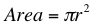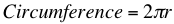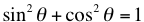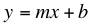Math Help: Formulas to Remember

09/21/2015

Math Formulas You Want to Know

As you advance in math, you will find certain operations and concepts will repeat and keep coming up. Here are some formulas that will help you get through your math problems quickly.

Circle FormulasThe area of a circle is the square of the radius times π.Multiply π times the diameter, or twice the radius, of a circle. When working with arcs, just remember that an arc is a percentage of a circle. Since there are 360 degrees in a circle, first calculate the percentage of the whole circle the arc represents. If the arc is 90 degrees, it is 90/360=1/4 or 25%. Multiply the area or circumference of the whole circle by this percentage to find the area or circumference of the arc.

SOHCAHTOA

To find the sine, cosine, and tangent of an angle, remember SOHCAHTOA. Sine= Opposite/Hypotenuse Cosine= Adjacent/Hypotenuse Tangent=Opposite/Adjacent Opposite is the side of the triangle directly opposite the angle in question. Adjacent is the side of the triangle next to the angle that is not the hypotenuse. The hypotenuse is the longest side of the triangle. It is also useful to know the reciprocals. Cosecant is the reciprocal of sine= Hypotenuse/Opposite Secant is the reciprocal of cosine= Hypotenuse/Adjacent Cotangent is the reciprocal of tangent= Adjacent/Opposite And finally, the square of an angles sine plus the square of an angle's cosine is 1 and the tangent is the quotient of the sine and cosine.Slope FormulaTo determine the slope of a line, choose two points on the line and divide the rise (how much the line goes up or down) by the run (how much the line goes left to right).

Slope-Intercept FormulaIn this formula, m is the slope and b is the y-intercept. If you get an equation that is not in this format, you will want to re-write it so that it is. It makes graphing a line much easier.

For Tutoring in Glendale, AZ

The Tutoring Center in Glendale, AZ offers one-to-one tutoring help in math, reading, writing, and more. Visit us online to find out more and call 623-566-6630 to schedule a free diagnostic assessment.

623-566-6630

FREE CONSULTATION

Schedule your Free Diagnostic Assessment Today!